Thermodynamics and Propulsion

17.1 The Reynolds Analogy

We describe the physical mechanism for the heat transfer coefficient in a turbulent boundary layer because most aerospace vehicle applications have turbulent boundary layers. The treatment closely follows that in Eckert and Drake (1959). Very near the wall, the fluid motion is smooth and laminar, and molecular conduction and shear are important. The shear stress,, at a plane is given by(where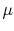is the dynamic viscosity), and the heat flux by. The latter is the same expression that was used for a solid. The boundary layer is a region in which the velocity is lower than the free stream as shown in Figures 17.2 and 17.3. In a turbulent boundary layer, the dominant mechanisms of shear stress and heat transfer change in nature as one moves away from the wall.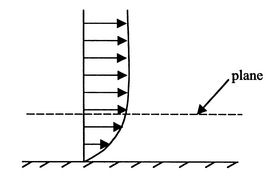As one moves away from the wall (but still in the boundary layer), the flow is turbulent. The fluid particles move in random directions and the transfer of momentum and energy is mainly through interchange of fluid particles, shown schematically in Figure 17.4.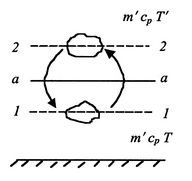With reference to Figure 17.4, because of the turbulent velocity field, a fluid mass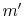penetrates the planeper unit time and unit area. In steady flow, the same amount crossesfrom the other side. Fluid moving up transports heat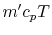. Fluid moving down transportsdownwards. If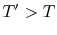, there is a turbulent downwards heat flow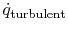, given by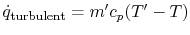, that results.

Fluid moving up also has momentumand fluid moving down has momentum. The net flux of momentum down per unit area and time is therefore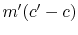. This net flux of momentum per unit area and time is a force per unit area or stress, given by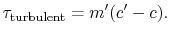(17..3)

Based on these considerations, the relation between heat flux and shear stress at planeis(17..4)

or (again approximately)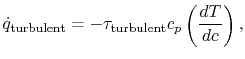(17..5)

since the locations of planes 1-1 and 2-2 are arbitrary.

For the laminar region, the heat flux towards the wall isand dividing by the expression for the shear stress,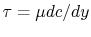, yields(17..6)

The same relationship is applicable in laminar or turbulent flow if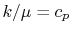or, expressed slightly differently,(17..7)

where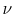is the kinematic viscosity, andis the thermal diffusivity.

The quantityis known as the Prandtl number (), after the man who first presented the idea of the boundary layer and was one of the pioneers of modern fluid mechanics. For gases, Prandtl numbers are in fact close to unity and for air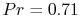at room temperature. The Prandtl number varies little over a wide range of temperatures: approximately 3% from 300-2000 K.

We want a relation between the values at the wall (at which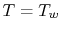and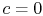) and those in the free stream. To get this, we integrate the expression forfrom the wall to the free stream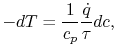(17..8)

where the relation between heat transfer and shear stress has been taken as the same for both the laminar and the turbulent portions of the boundary layer. The assumption being made is that the mechanisms of heat and momentum transfer are similar. Equation (17.8) can be integrated from the wall to the freestream (conditions at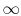''):(17..9)

where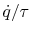and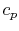are assumed constant.

Carrying out the integration yields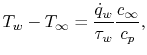(17..10)

where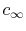is the velocity andis the specific heat. In Equation (17.10),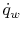is the heat flux to the wall and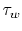is the shear stress at the wall. The relation between skin friction (shear stress) at the wall and heat transfer is thus(17..11)

The quantity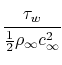is known as the skin friction coefficient and is denoted by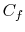. The skin friction coefficient has been tabulated (or computed) for a large number of situations. If we define a non-dimensional quantity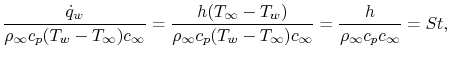(17..12)

known as the Stanton Number, we can write an expression for the heat transfer coefficient,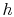as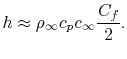(17..13)

Equation (17.13) provides a useful estimate of, or, based on knowing the skin friction, or drag. The direct relationship between the Stanton Number and the skin friction coefficient is(17..14)

The relation between the heat transfer and the skin friction coefficient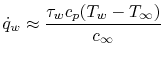(17..15)

is known as the Reynolds analogy between shear stress and heat transfer. The Reynolds analogy is extremely useful in obtaining a first approximation for heat transfer in situations in which the shear stress is known.''

An example of the use of the Reynolds analogy is in analysis of a heat exchanger. One type of heat exchanger has an array of tubes with one fluid flowing inside and another fluid flowing outside, with the objective of transferring heat between them. To begin, we need to examine the flow resistance of a tube. For fully developed flow in a tube, it is more appropriate to use an average velocityand a bulk temperature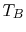. Thus, an approximate relation for the heat transfer is(17..16)

The fluid resistance (drag) is all due to shear forces and is given by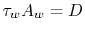, whereis the tube wetted'' area (perimeterlength). The total heat transfer,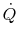, is, so that(17..17)

The power,, to drive the flow through a resistance is given by the product of the drag and the velocity,, so that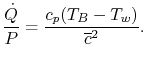(17..18)

The mass flow rate is given by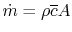, whereis the cross sectional area. For a given mass flow rate and overall heat transfer rate, the power scales asor as, i.e.,(17..19)

Equations (17.18) and (17.19) show that to decrease the power dissipated, we need to decrease, which can be accomplished by increasing the cross-sectional area. Two possible heat exchanger configurations are sketched in Figure 17.5; the one on the right will have a lower loss.To recap, there is an approximate relation between skin friction (momentum flux to the wall) and heat transfer called the Reynolds analogy that provides a useful way to estimate heat transfer rates in situations in which the skin friction is known. The relation is expressed by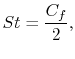or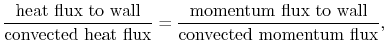or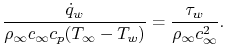The Reynolds analogy can be used to give information about scaling of various effects as well as initial estimates for heat transfer. It is emphasized that it is a useful tool based on a hypothesis about the mechanism of heat transfer and shear stress and not a physical law.

Muddy Points

What is the analogy'' that we are discussing? Is it that the equations are similar? (MP 17.2)

In what situations does the Reynolds analogy not work?'' (MP 17.3)

UnifiedTP